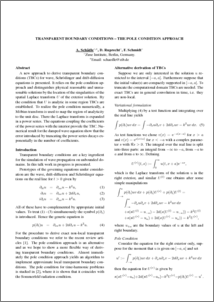Repository: Freie Universität Berlin, Math Department

# Transparent boundary conditions - the pole condition approach

Schaedle, A. and Ruprecht, D. and Schmidt, F. (2007) Transparent boundary conditions - the pole condition approach. In: The 8th International Conference on Mathematical and Numerical Aspects of Waves, 23rd - 27th July 2007, Reading UK.Preview

58kB

## Abstract

A new approach to derive transparent boundary conditions (TBCs) for wave, Schr¨odinger and drift-diffusion equations is presented. It relies on the pole condition approach and distinguishes physical reasonable and unreasonable solutions by the location of the singularities of the spatial Laplace transform U of the exterior solution. By the condition that U is analytic in some region TBCs are established. To realize the pole condition numerically, a Möbius transform is used to map the region of analyticity to the unit disc. There the Laplace transform is expanded in a power series. The equations coupling the coefficients of the power series with the interior provide the TBC. Numerical result for the damped wave equation show that the error introduced by truncating the power series decays exponentially in the number of coefficients.

Item Type: Conference or Workshop Item (Paper) N. Biggs et.al. editors Mathematical and Computer Sciences > Mathematics > Applied Mathematics Department of Mathematics and Computer Science > Institute of Mathematics > Geophysical Fluid Dynamics Group 795 Ulrike Eickers 20 Nov 2009 15:11 03 Mar 2017 14:40

Repository Staff Only: item control page